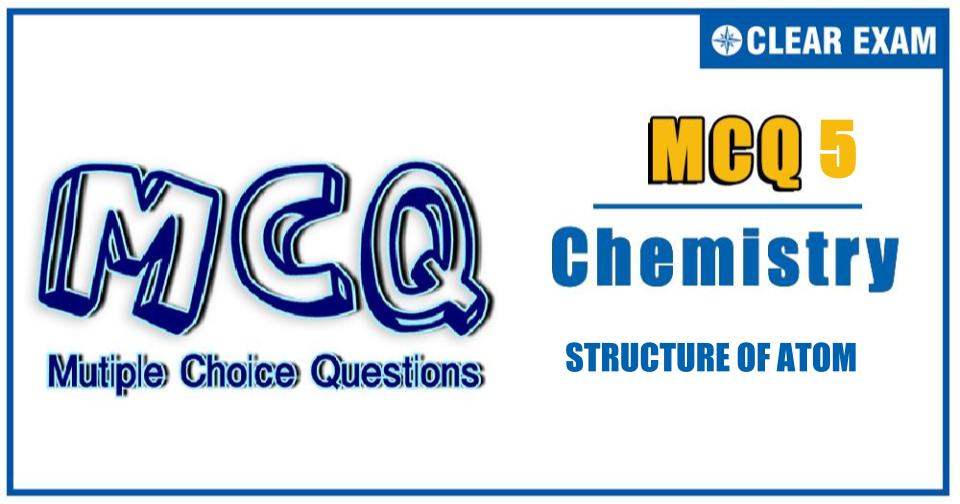## Chemistry Aptitude Quiz

IIT JEE exam which consists of JEE Main and JEE Advanced is one of the most important entrance exams for engineering aspirants. The exam is held for candidates who are aspiring to pursue a career in the field of engineering and technical studies. Chemistry is important because everything you do is chemistry! Even your body is made of chemicals. Chemical reactions occur when you breathe, eat, or just sit there reading. All matter is made of chemicals, so the importance of chemistry is that it's the study of everything..

Q1. The wave mechanical model of an atom is based upon which of the following equations?
•  Schrodinger’s equation
•  De Broglie’s equation
•  Heisenberg’s uncertainty principle
•  All the above
Solution
Part D

Q2.The correct set of four quantum numbers for the valence (outermost) electron of rubidium (Z=37) is
•  5,0,0,+1⁄2
•  5,1,0,+1⁄2
•  5,1,1,+1⁄2
•  6,0,0,+1⁄2
Solution
(b)
Rb(z=37)
[Kr]_36 5s^1
For the last electron:
n=5,l=0,m=0,s=1⁄2

Q3.  The radiation that produces the greatest number of ions as it passes through matter is
•  α
•  γ
•  β^-
•  β^+
Solution
Part A
Q4.   The decay of a radioactive element follows first order kinetics. Thus,
•  Half-life period = a constant/K, where K is decay constant
•   The rate of decay is independent of temperature
•   The rate can be altered by changing chemical conditions
•  The element will be completely transformed into new element after expiry of two half-life period
Solution

(a)
t_(1/2)=0.693/K
Q5.After three half lives, the percentage of fraction of amount left is
•  6.35
•  12.5
•  50
•  75
Solution
(b)
Given n=3, we know that N=(1/2)^n N_0
∴(N/N_0 )=(1/2)^n=1/8 Or N/N_0 %=1/8×100=12.5%

Q6. The SI unit of radioactivity is
•  Curie
•  Micro-curie
• Rutherford
•  Becquerel
Solution
Part A

Q7.Which nuclear reaction is not balanced?
•   _5^10 B+ _2^4 He→ _7^13 N+ _0^1 n
•   _92^238 U+ _2^4 He→ _95^241 Am+ _0^1 n
•   _18^40 Ar+ _1^1 H→ _19^40 K+ _0^1 n
•   _7^14 N+ _2^4 He→ _8^17 O+ _1^1 H
Solution
(b)
_92^238 U+ _2^4 He→ _95^241 Am+ _0^1 n
LHS     RHS
Mass number   242      242   balanced
Atomic number   94      95   unbalanced

Q8.Atoms with the same mass number but having different nuclear charges are called
•  Isotopes
•  Isobars
•  Isochors
•  Isotones
Solution
(b)
Isobars have different mass number

Q9.The number of radial nodes of 3s and 2p-orbitals are respectively
•  2, 0
•  0, 2
•  1, 2
•  2, 11
Solution
(a)

Q10. C-14 has a life of 5760 years. 100 mg of sample containing C-14 is reduced to 25 mg in
•  11520 years
•  2880 years
•  1440 years
• 17280 years
Solution
Part A#### Written by: AUTHORNAME

AUTHORDESCRIPTION## Want to know more

Please fill in the details below:

## Latest NEET Articles\$type=three\$c=3\$author=hide\$comment=hide\$rm=hide\$date=hide\$snippet=hide

Name

ltr
item
BEST NEET COACHING CENTER | BEST IIT JEE COACHING INSTITUTE | BEST NEET & IIT JEE COACHING: STRUCTURE OF ATOM Quiz-5
STRUCTURE OF ATOM Quiz-5
https://1.bp.blogspot.com/-omEFcsyZylo/YOWtKsnC8cI/AAAAAAAA31Q/qrUZrWSe0qUafCgXcZTlpjgbD2iEAU0EgCLcBGAsYHQ/s960/Quiz%2BImage%2B20%2B%252829%2529.jpg
https://1.bp.blogspot.com/-omEFcsyZylo/YOWtKsnC8cI/AAAAAAAA31Q/qrUZrWSe0qUafCgXcZTlpjgbD2iEAU0EgCLcBGAsYHQ/s72-c/Quiz%2BImage%2B20%2B%252829%2529.jpg
BEST NEET COACHING CENTER | BEST IIT JEE COACHING INSTITUTE | BEST NEET & IIT JEE COACHING
https://www.cleariitmedical.com/2021/07/structure-of-atom-quiz-5.html
https://www.cleariitmedical.com/
https://www.cleariitmedical.com/
https://www.cleariitmedical.com/2021/07/structure-of-atom-quiz-5.html
true
7783647550433378923
UTF-8

STAY CONNECTED